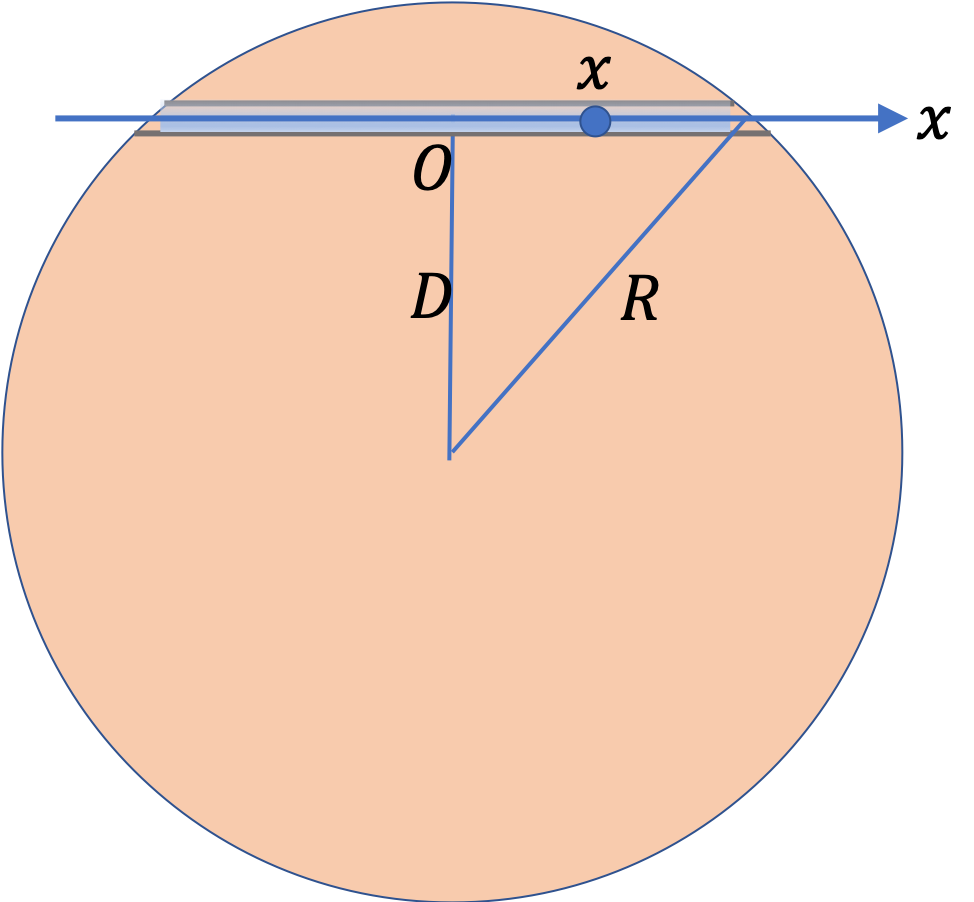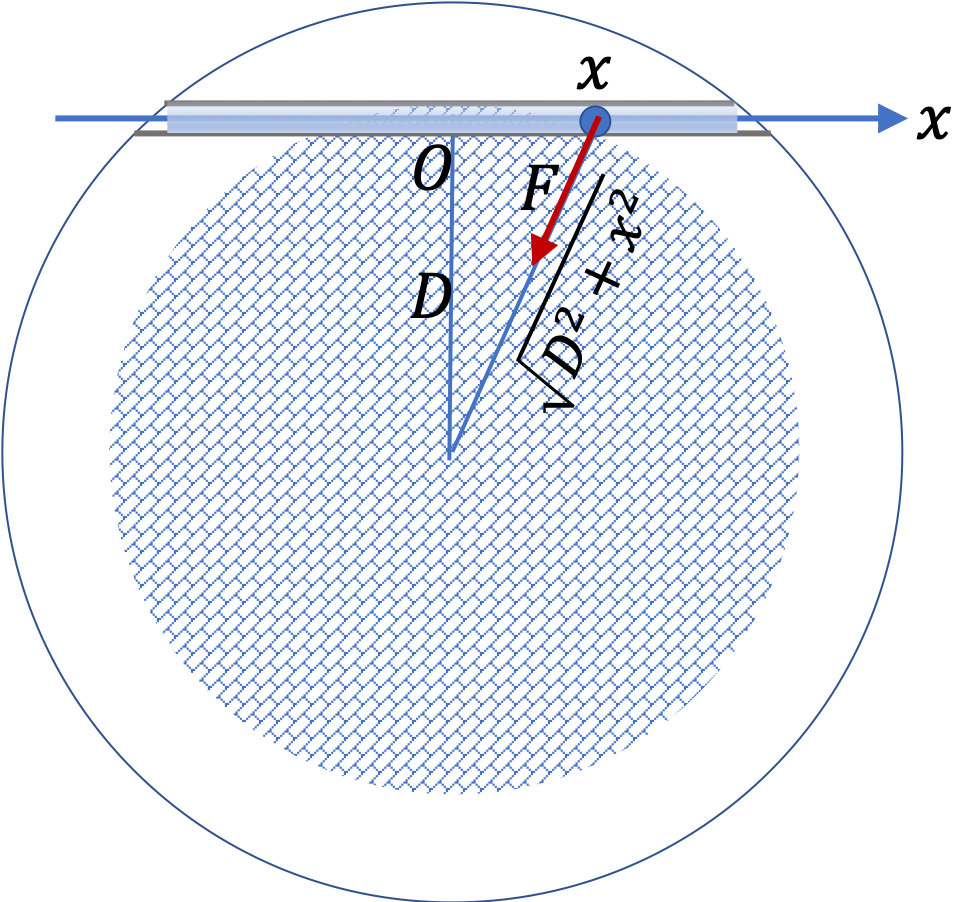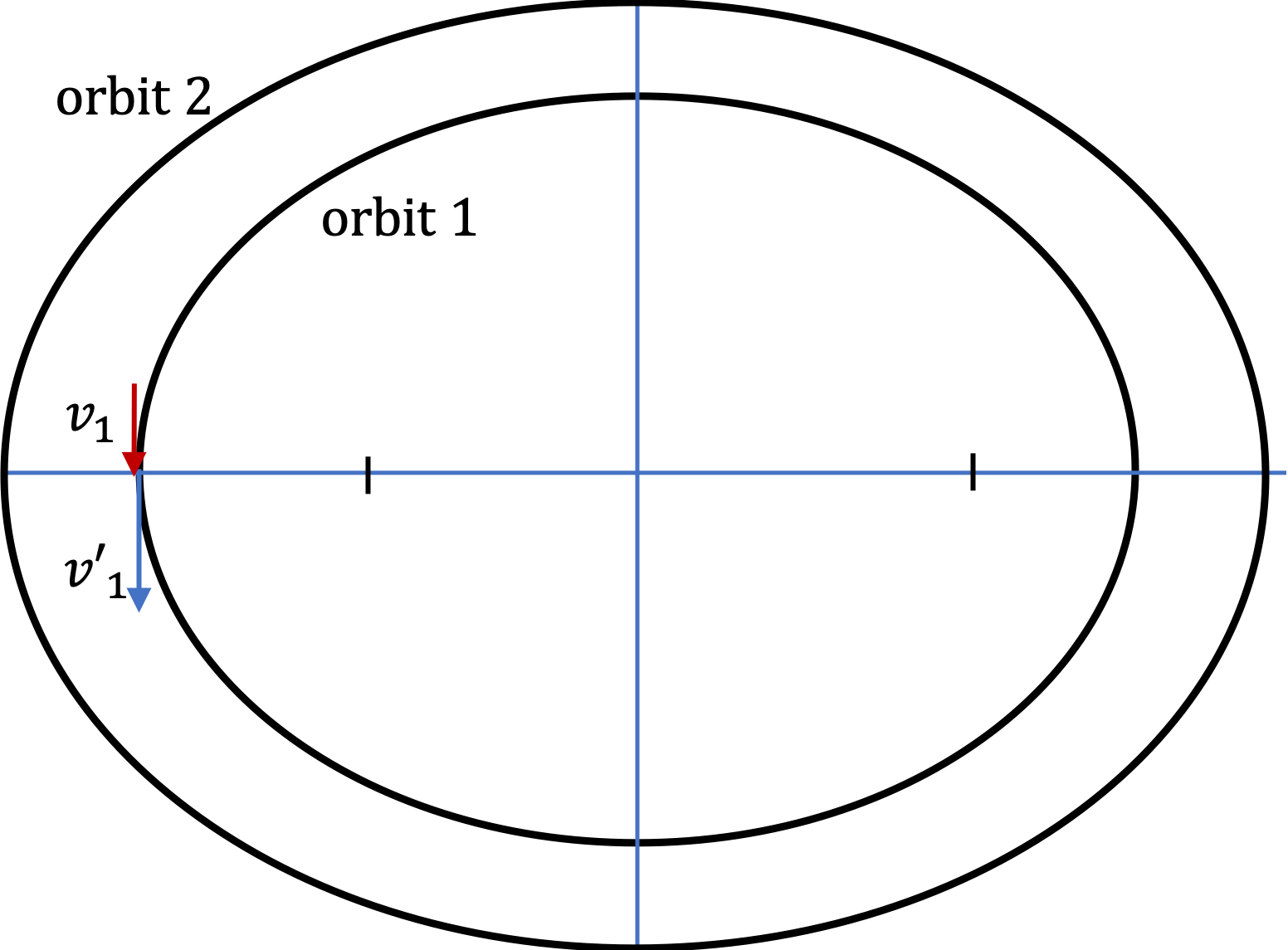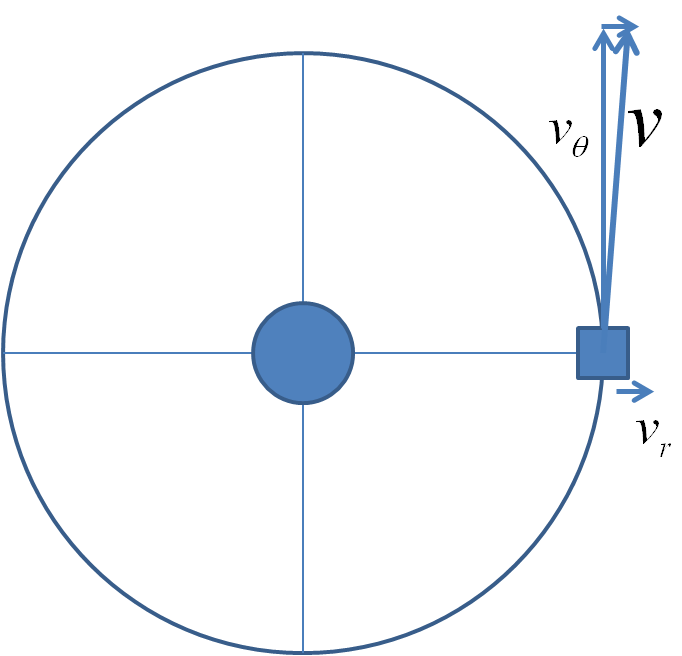## Section12.10Gravitation Bootcamp

### Subsection12.10.10Miscellaneous

A tunnel is dug through Earth at a vertical distance $D$ from the center of the Earth. A small spherical ball is dropped in the tunnel. Assume no friction between the ball and the tunnel. Suppose $x$ axis is along the tunnel wth origin at the midpoint.(a) Find the component of the force of gravity along the tunnel.

(b) What will be the equation of motion of the ball?

(c) Your equation of motion should turn out to be the same as the equation of motion of a simple harmonic motion. (i) From your equation of motion, read off the formula for the angular frequency of the back-and-forth oscillation of the ball. (ii) Also, find numerical value of the period of the oscillations.

(d) If the ball was dropped at rest, where will the ball be at an arbitrary instant and how fast will it be traveling?

Hint

(a) The magnitude of the force by Earth will be from the mass that is between the center and position of the ball.

(a) $-\dfrac{ G_N M m}{R^3}\, x\text{,}$ (b) $m a_x = -\dfrac{ G_N M m}{R^3}\, x \text{,}$ (c) (i) $\omega = \sqrt{ \dfrac{ G_N M}{R^3} }\text{,}$ (c) (ii) $5,050\text{ sec}$ (d) $x = \sqrt{R^2 - D^2}\, \cos\,\omega t\text{,}$ $v = -\omega\, \sqrt{R^2 - D^2}\, \sin\,\omega t \text{.}$

Solution 1 (a)

(a) The magnitude of the force on the ball will be from the mass of Earth that is between the center of Earth and the position if tha ball. Suppose the ball is at $x$coordinate $x\text{.}$ Then, the ball is at a radial distance $\sqrt{ D^2 + x^2}$ from the center.The mass of the Earth we need for the force formula is

\begin{equation*} \dfrac{M}{\frac{4}{3}\pi R^3}\ \frac{4}{3}\pi \left(\sqrt{ D^2 + x^2} \right)^3. \end{equation*}

Let's denote this by symbol $M^{\prime}_E\text{.}$

The magnitude of the force on the sphere will be

\begin{align*} F \amp = G_N \dfrac{ m M^{\prime}_E}{ D^2 + x^2 }, \\ \amp = G_N \dfrac{Mm}{R^3}\, \sqrt{ D^2 + x^2}. \end{align*}

We need $x$ component of this force.

\begin{equation*} F_x = - F\, \dfrac{x}{ \sqrt{ D^2 + x^2} } = -\dfrac{ G_N M m}{R^3}\, x. \end{equation*}
Solution 2 (b)

(b) Equation of motion will be the $x$ component of $m\vec a = \vec F\text{.}$

\begin{equation*} m a_x = -\dfrac{ G_N M m}{R^3}\, x. \end{equation*}
Solution 3 (c)

(c) Equation of motion shows that acceleration is proportional to the displacement $x$ and is in the opposite direction. This is same as equation of motion for a simple harmonic motion.

Letting

\begin{equation*} \omega^2 = \dfrac{ G_N M}{R^3}. \end{equation*}

we get

\begin{equation*} a_x = -\omega^2\, x. \end{equation*}

(i) That is, the angular frequency is

\begin{equation*} \omega = \sqrt{ \dfrac{ G_N M}{R^3} }. \end{equation*}

(ii) The period of oscillaions is

\begin{equation*} T = \dfrac{2\pi}{\omega} = 2\pi \sqrt{ \dfrac{R^3}{G_N M} }. \end{equation*}

Putting in the numbers for Earth we get

\begin{equation*} T = 5,050\text{ sec}. \end{equation*}
Solution 4 (d)

(d) The analogy with simple harmonic motion can be used to solve the equation of motion of the ball to yield the following for position and velocity.

\begin{align*} x \amp = A \cos\,\omega t + B \sin\,\omega t,\\ v \amp = -\omega A \sin\,\omega t + \omega B \cos\,\omega t. \end{align*}

Starting with zero velocity means, we have $B=0\text{.}$ Starting at $x=\sqrt{R^2 - D^2}$ gives

\begin{equation*} A = \sqrt{R^2 - D^2}. \end{equation*}

Therefore, position and velocity at arbitrary instant are

\begin{align*} x \amp = \sqrt{R^2 - D^2}\, \cos\,\omega t,\\ v \amp = -\omega \sqrt{R^2 - D^2} \sin\,\omega t, \end{align*}

with

\begin{equation*} \omega = \sqrt{ \dfrac{ G_N M}{R^3} }. \end{equation*}

We want to send a spaceship of mass $3000\text{ kg}$ is from Earth to Venus. Assume the orbits of the Earth and the Venus around the Sun to be approximately circular.

(a) What is the minimum energy required for the transfer?

(b) The most efficient transfer from one circular orbit to another circular orbit is achieved by what is called Hohmann transfer. In Hohmann transfer, we use an elliptical orbit as an intermediary with the smaller and larger circles located at perihelion and aphelion of the ellipse.

The speed of the satellite must be increased at point Q and reduced at point P for the transfer. Find the changes in speed required at points P and Q.

Note that the spaceship will have speeds of Earth and Venus while in their orbits. Ignore the gravitational pulls of the Earth and the Venus on the spaceship.

Hint

(a) Use equations of circular motion and conservation of energy, (b) Use angular momentum and energy conservations.

(a) $\Delta E = 5.12 \times 10^{11} \text{ J} \text{,}$ (b) See solution.

Solution 1 (a)

(a) We are assuming that the orbits of Earth and Venus are circular orbits. Since the radius of the orbit of Venus is smaller than that ofg Earth, you need less energy to be in the orbit of Venus. Let us denote by $E_1$ and $E_2\text{,}$ the energy of the space ship when it is in Earth's orbit around the Sun and it is in Venus's orbit around the Sun, respectively. Let $r_1$ is the distance of Earth from Sun and $r_2$ the distance of Venus. Let $v_1$ be the speed of the ship when in Earth orbit and $v_2$ the speed when in the Venus orbit. Let $M_S$ the mass of the Sun.

Applying work-energy theorem on the spaceship will give us

\begin{equation} \Delta E = E_1 - E_2,\label{pr-hohmann-transfer-a-1}\tag{12.10.1} \end{equation}

with the following expressions

\begin{align*} E_1 \amp \dfrac{1}{2} m v_1^2 - \dfrac{G_N M_S m}{r_1},\\ E_2 \amp \dfrac{1}{2} m v_2^2 - \dfrac{G_N M_S m}{r_2}. \end{align*}

In case of circular orbit, we also have

\begin{equation*} m \dfrac{v_1^2}{r_1} = \dfrac{G_N M_S m}{r_1^2}, \end{equation*}

and similarly for 2. Using these in the conservation equations above we get

\begin{align*} E_1 \amp = - \dfrac{G_N M_S m}{2r_1},\\ E_2 \amp = - \dfrac{G_N M_S m}{2r_2}. \end{align*}

Using these in Eq. (12.10.1) we get

\begin{equation*} \Delta E = \dfrac{1}{2} G_N M_S m\, \left( \dfrac{r_1 -r_2 }{r_1 r_2} \right). \end{equation*}

Now, we numerical values to get a numerical answer.

\begin{align*} m \amp = 3000\text{ kg},\ \ M_S = 2.0\times 10^{30}\text{ kg}, \\ r_1 \amp = 1.496\times 10^{11}\text{ m},\ \ r_2 = 1.082\times 10^{11}\text{ m}, \end{align*}

These numbers give the value of $\Delta E$ to be

\begin{equation*} \Delta E = 5.12 \times 10^{11} \text{ J}. \end{equation*}
Solution 2 (b)

(b) Let $v_P$ and $v_Q$ be the speeds of the space ship when it in its elliptical orbit under the influence of Sun only. The elliptical motion has both conservation of angular momentum and conservation of energy.

Conservation of angular momentum between P and Q in the elliptical motion gives

\begin{equation} m v_P r_1 = m v_Q r_2.\label{pr-hohmann-transfer-b-ang-mom}\tag{12.10.2} \end{equation}

Conservation of energy between P and Q in the elliptical motion gives

\begin{equation} \dfrac{1}{2} m v_P^2 - \dfrac{G_N M m}{r_1} = \dfrac{1}{2} m v_Q^2 - \dfrac{G_N M m}{r_2}.\label{pr-hohmann-transfer-b-energy}\tag{12.10.3} \end{equation}

We can solve these two equations for $v_P$ after eliminating $v_Q\text{.}$

\begin{equation*} v_P = \sqrt{ 2G_N M \left( \dfrac{1}{r_2} - \dfrac{1}{r_1} \right) / \left( \dfrac{r_1^2}{r_2^2} -1 \right) }. \end{equation*}

We will then use Eq. (12.10.2) to get $v_Q\text{.}$

\begin{equation*} v_Q = \dfrac{r_1}{r_2}\, v_P. \end{equation*}

Therefore, we need the following change in speeds at the two points: from $v_1$ to $v_P$ slowing down and from $v_2$ to $v_Q$ speeding up.

\begin{align*} \amp \Delta v_P = \left|v_1 - v_P \right|, \\ \amp \Delta v_Q = \left|v_2 - v_Q \right|. \end{align*}

A satellite is in an elliptical orbit about Earth with a minimum altitude of $1,500\text{ km}$ and a maximum altitude of $8,000\text{ km}\text{.}$ Data: radius of Earth = $6.378\times 10^{6}\text{ km}\text{.}$

In one of the flights, its engine is fired when it is at the closest approach point so that its speed increases by 10%.

What is the minimum altitude reached in its new elliptical orbit? You can assume the speed increased over a very short period of time.Hint

Use energy in orbit equation to find $v\text{.}$ With $v'=1.1\,v\text{,}$ use energy in oribit to find eccentricity of the new orbit.

$2.5\times 10^7\ \text{m}\text{.}$

Solution

From the given description we have

\begin{align*} \amp r_\text{min} = R_E + 1.5\times 10^6\ \text{m} = 6.87\times 10^{6}\ \text{m}.\\ \amp r_\text{max} = R_E + 8.0\times 10^6\ \text{m} = 14.37\times 10^{6}\ \text{m}. \end{align*}

The energy of the satellite in an elliptical orbit is given in terms of the semi-major axis. Let $M$ be the mass of the Earth.

\begin{equation*} E = -\frac{G_N M m}{r_\text{max} + r_\text{min}} \end{equation*}

Since the mass of the satellite is not given in the problem, let us write this for per unit mass.

\begin{equation*} \frac{E}{m} = -\frac{G_N M }{r_\text{max} + r_\text{min}} = -1.87\times 10^7\ \text{J/kg}. \end{equation*}

Equating this to the sum of the kinetic and potential energies when at the closest point gives, again writing per unit mass,

\begin{equation*} \frac{1}{2} v^2 - \frac{G_N M}{r_\text{min}} = -1.87\times 10^7, \end{equation*}

which can be solved for $v$ giving

\begin{equation*} v = 8,870\ \text{m/s}. \end{equation*}

Now, the speed increases by 10\%. The new speed $v'$ will be

\begin{equation*} v' = 1.1 \times 8870 = 9,760\ \text{m/s}. \end{equation*}

Therefore we have per unit mass

\begin{align*} \text{new energy}\ \amp =\ \text{old energy} - \text{old KE} + \text{new KE}\\ \amp = -1.87\times 10^7 - \frac{1}{2} \times 8870^2+ \frac{1}{2} \times 9,760^2 \\ \amp = -1.04 \times 10^7\ \text{J/kg}. \end{align*}

But the satellite will still have the same $r_\text{min}$ given by $6.87\times 10^{6}\text{ m}\text{.}$ With this new energy and the $r_\text{min}$ we can deduce the eccentricity $e_\text{new}$ of the new orbit. From the eccentricity and $r_\text{min}$ we can calculate the new $r_\text{max}^{new}\text{.}$ Let us implement this procedure. From

\begin{equation*} \frac{r_\text{min}}{1-e} = \frac{G_N M m}{2E}, \end{equation*}

we obtain

\begin{equation*} e = 1 + \frac{2E r_\text{min}}{G_N M m } = 0.641. \end{equation*}

The new $r_\text{max}$ will be

\begin{equation*} r_\text{max}^{new} = \left( \frac{1+e_\text{new}}{1-e_\text{new}}\right) r_\text{min} = 3.14\times 10^7\ \text{m}. \end{equation*}

We now subtract the radius of the Earth to get the new altitude $H_\text{new}\text{.}$

\begin{equation*} H_\text{new} = r_\text{max}^{new} -R_E = 2.5\times 10^7\ \text{m}. \end{equation*}

A space station of mass $m$ moves in a circular orbit of radius $R$ around Jupiter (mass $M$). A rocket of mass $\Delta m$ is fired radially inward towards the center of Jupiter from the space station which causes the space station to “instantaneously” acquire a radial velocity $v_r$ in addition to the tangential velocity $v_{\theta}$ it had before the rocket firing. As a result, the space station is thrown into an elliptical orbit.

(a) Find the semimajor axis and eccentricity of the elliptical orbit.

(b) What would have happened if the rocket was fired tangentially to the circular orbit with relative speed $u$ towards the front?

Hint

(a) 􏱐 During the inward firing angular momentum will be conserved but energy will increase. Setting up these two conditions will give you information about $r_\text{min}$ and $r_\text{max}$ of the resulting elliptical orbit. (b) In this case both angular momentum and energy will be conserved.

(a) $\text{.}$ (b) $\text{.}$

Solution

(a) The physical situation as a result of thrust is illustrated in this figure. Since the thrust is radial, the angular momentum about the center or focus of the orbit will remain unchanged. However, the energy will change.We can combine the information in the new energy and the new expression for the angular momentum to obtain the closest and farthest approaches of the space station in the elliptic orbit. Adding the two will give the length of the major axis.

Let $v_0$ be the speed of the space station before firing. Let $r_1$ and $r_2$ be the closest and furthest distance from the focus of the orbital path and $v$ be the speed of the satellite after the firing. From the given data we have

\begin{align*} \amp v_0 = v_{\theta}\\ \amp v^2 = v_{\theta}^2 + v_r^2 = v_0^2 + v_r^2. \end{align*}

Let us denote by a prime on $E$ and $l$ for the energy and angular momentum after the firing and same symbols wihtout the prime for the quantities before the firing. Ignoring the energy in the portion that left the space station, we have

\begin{align} \amp l'= l = m v_0 R. \label{eq-ch11-pr9-1}\tag{12.10.4}\\ \amp E' = E + \frac{1}{2} m v_r^2, \label{eq-ch11-pr9-2}\tag{12.10.5} \end{align}

where $E$ is the energy before the firing,

\begin{equation*} E = -\frac{G_N M m }{2R}, \end{equation*}

which includes the kinetic energy $\frac{1}{2}m v_0^2\text{.}$ Now, when the station is in the elliptical orbit we can write the angular momentum and energy in terms of the parameters of the orbit as follows.

\begin{align} \amp l'=m r_1 v_1, \label{eq-ch11-pr9-3}\tag{12.10.6}\\ \amp E' = -\frac{G_N M m}{r_1 + r_2}, \label{eq-ch11-pr9-4}\tag{12.10.7} \end{align}

where $v_1$ is the speed of the station when it is at the closest point in the elliptical orbit. We can write the energy $E'$ also in terms of the parameters $v_1$ and $r_1$ as

\begin{equation} E' = \frac{1}{2} m v_1^2 - \frac{G_N M m}{r_1}. \label{eq-ch11-pr9-5}\tag{12.10.8} \end{equation}

From Eqs. (12.10.4) - (12.10.8) we can obtain the expressions for $r_1$ and $r_2$ by eliminating $v_1$ from these equations.

(b) When the space station is fired tangentially both the energy and angular momentum changes. The exercise is left for the student to complete.